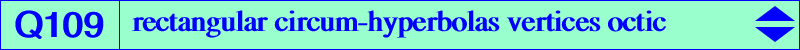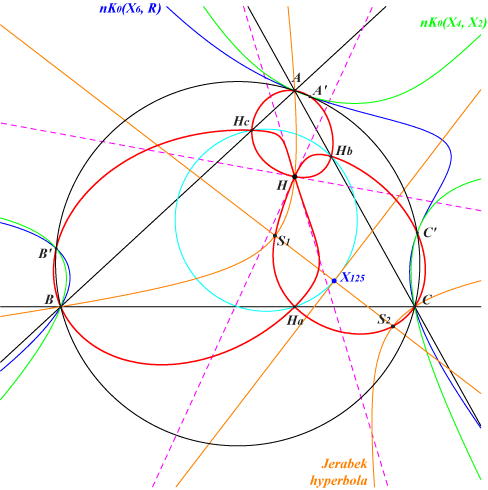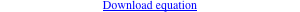too complicated to be written here. Click on the link to download a text file.X(4) vertices of the orthic triangleQ109 is the locus of vertices of rectangular circum-hyperbolas. Recall that their centers lie on the nine point circle. Q109 is a quadricircular circum-octic passing through H and the vertices Ha, Hb, Hc of the orthic triangle. A, B, C, H are triple points and Ha, Hb, Hc are double points with perpendicular tangents. The tangents at H are parallel to the asymptotes of nK0(X25, X4). Q109 meets the circumcircle again at three points A', B', C' which also lie on nK0(X4, X2) and nK0(X6, R) where R is the intersection of the lines X2-X216 , X3-X112 , X6-X22 , X20-X39 , etc. SEARCH = 0.223376407533024. R is X(22240) in ETC.